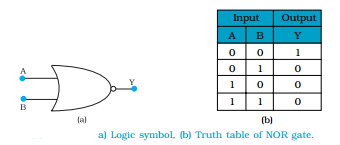Logic gates

A gate is a digital circuit that follows curtain logical relationship between the input and output voltages. Therefore, they are generally known as logic gates — gates because they control the flow of information. The five common logic gates used are NOT, AND, OR, NAND, NOR

These logic gates can be realized using semiconductor devices.

NOT gate:

This is the most basic gate, with one input and one output. It produces a ‘1’ output if the input is ‘0’ and vice-versa. That is, it produces an inverted version of the input at its output.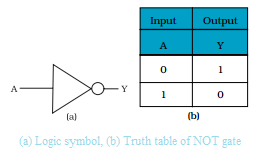OR gate:

An OR gate has two or more inputs with one output. The output Y is 1 when either input A or input B or both are 1s, that is, if any of the input is high, the output is high.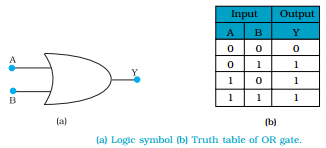AND gate:

An AND gate has two or more inputs and one output. The output Y of AND gate is 1 only when input A and input B are both 1.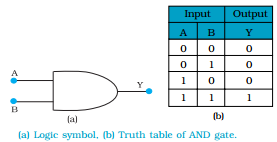NAND gate:

This is an AND gate followed by a NOT gate. If inputs A and B are both ‘1’, the output Y is not ‘1’. The gate gets its name from this NOT AND behavior.
NAND gates are also called Universal Gates since by using these gates you can realize other basic gates like OR, AND and NOT.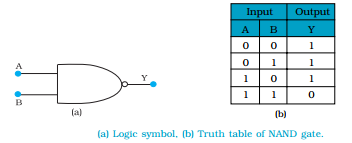NOR gate:

It has two or more inputs and one output. A NOT- operation applied after OR gate gives a NOT-OR gate (or simply NOR gate). Its output Y is ‘1’ only when both inputs A and B are ‘0’, i.e., neither one input nor the other is ‘1’.

NOR gates are considered as universal gates because you can obtain all the gates like AND, OR, NOT by using only NOR gates